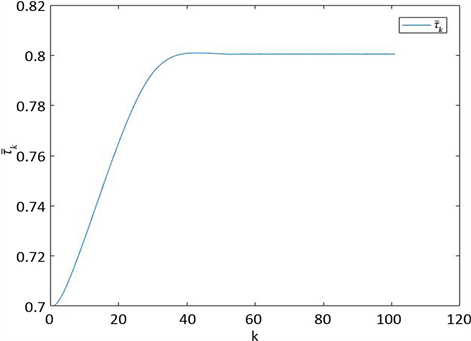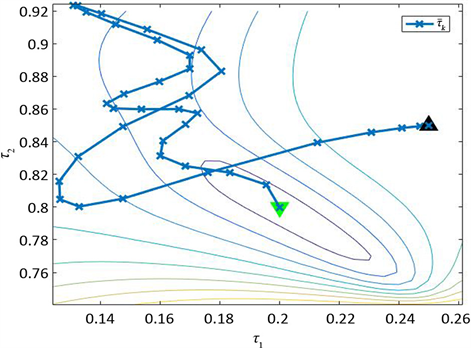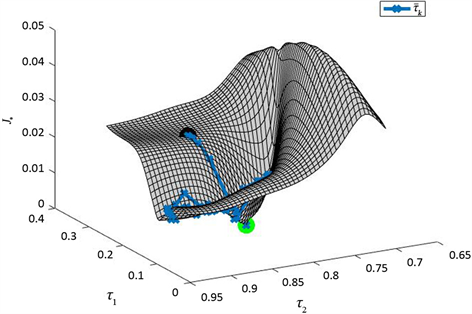# 基于梯度方法的线性多延时系统参数辩识Parameter Identification of Linear Multi-Delay System Based on Gradient Method

DOI: 10.12677/PM.2021.114071, PDF, HTML, XML, 下载: 23  浏览: 96

Abstract: For a class of linear systems with multiple delays, a new parameter identification algorithm based on gradient and least square method is proposed. Firstly, the joint estimation problem of coefficient matrix and time delay is transformed into the optimization problem which only estimates time delay. Then, the estimated value of time delay is obtained based on momentum gradient method. Finally, by using the least square solution of matrix equation, the estimated value of coefficient matrix is obtained. The algorithm has good identification effect.

1. 引言

2. 问题描述

$\left\{\begin{array}{ll}\frac{\text{d}}{\text{d}t}x\left(t\right)=\underset{i=0}{\overset{N}{\sum }}\text{ }\text{ }{A}_{i}x\left(t-{\tau }_{i}\right)+\underset{i=0}{\overset{N}{\sum }}\text{ }\text{ }{B}_{i}u\left(t-{\tau }_{i}\right),\hfill & t\in \left[0,T\right];\hfill \\ x\left(t\right)=\phi \left(t\right),\hfill & -\tau \le t\le 0.\hfill \end{array}$ (1)

$\Theta =\left[\begin{array}{cccc}{A}_{0}& {A}_{1}& \cdots & {B}_{N}\end{array}\right]$$\stackrel{¯}{\tau }={\left[\begin{array}{ccc}{\tau }_{1}& \cdots & {\tau }_{N}\end{array}\right]}^{\text{T}}$

$\begin{array}{c}{‖Q‖}_{F}=\sqrt{trace\left({\left[\begin{array}{ccc}{q}_{1}& \cdots & {q}_{n}\end{array}\right]}^{\text{T}}\left[\begin{array}{ccc}{q}_{1}& \cdots & {q}_{n}\end{array}\right]\right)}\\ =\sqrt{\underset{i=1}{\overset{m}{\sum }}\text{ }\text{ }{q}_{i}^{\text{T}}{q}_{i}}=\sqrt{\underset{i=1}{\overset{m}{\sum }}{‖{q}_{i}‖}_{2}^{2}}={‖{\left[\begin{array}{ccc}{q}_{1}^{\text{T}}& \cdots & {q}_{m}^{\text{T}}\end{array}\right]}^{\text{T}}‖}_{2}\end{array}$

$AXB=F,$

$\frac{\text{d}}{\text{d}t}{‖Q\left(t\right)‖}_{F}^{2}=\frac{\text{d}}{\text{d}t}tr\left({Q}^{\text{T}}\left(t\right)Q\left(t\right)\right)=tr\left(\frac{\text{d}}{\text{d}t}\left({Q}^{\text{T}}\left(t\right)Q\left(t\right)\right)\right)=2tr\left({Q}^{\text{T}}\left(t\right)\frac{\text{d}}{\text{d}t}Q\left(t\right)\right).$

$\begin{array}{c}\underset{\stackrel{¯}{\tau },\Theta }{min}J\left(\stackrel{¯}{\tau },\Theta \right)=\underset{l=1}{\overset{m}{\sum }}{‖x\left({t}_{l}\right)-x\left(0\right)-\left[\underset{i=0}{\overset{N}{\sum }}\text{ }\text{ }{A}_{i}{\int }_{0}^{{t}_{l}}\text{ }\text{ }x\left(t-{\tau }_{i}\right)\text{d}t+\underset{i=0}{\overset{N}{\sum }}\text{ }\text{ }{B}_{i}{\int }_{0}^{{t}_{l}}\text{ }\text{ }u\left(t-{\tau }_{i}\right)\text{d}t\right]‖}_{2}^{2}\\ =\underset{l=1}{\overset{m}{\sum }}{‖x\left({t}_{l}\right)-x\left(0\right)-\Theta \varphi \left(\stackrel{¯}{\tau },l\right)‖}_{2}^{2}.\end{array}$ (2)

$\varphi \left(\stackrel{¯}{\tau },l\right)={\left[\begin{array}{cccccc}{\int }_{0}^{{t}_{l}}\text{ }\text{ }{x}^{\text{T}}\left(t\right)\text{d}t& \cdots & {\int }_{0}^{{t}_{l}}\text{ }\text{ }{x}^{\text{T}}\left(t-{\tau }_{N}\right)\text{d}t& {\int }_{0}^{{t}_{l}}\text{ }\text{ }{u}^{\text{T}}\left(t\right)\text{d}t& \cdots & {\int }_{0}^{{t}_{l}}\text{ }\text{ }{u}^{\text{T}}\left(t-{\tau }_{N}\right)\text{d}t\end{array}\right]}^{\text{T}}.$ (3)

3. 算法的推导

3.1. 梯度的计算

$\stackrel{^}{\Theta }\left(\stackrel{¯}{\tau }\right)=\stackrel{˜}{X}{\Phi }^{\text{T}}\left(\stackrel{¯}{\tau }\right){\left(\Phi \left(\stackrel{¯}{\tau }\right){\Phi }^{\text{T}}\left(\stackrel{¯}{\tau }\right)\right)}^{-1}.$ (4)

$\stackrel{˜}{X}=\left[\begin{array}{ccc}x\left({t}_{1}\right)-x\left(0\right)& \cdots & x\left({t}_{m}\right)-x\left(0\right)\end{array}\right],$ (5)

$\Phi \left(\stackrel{¯}{\tau }\right)=\left[\begin{array}{ccc}\varphi \left(\stackrel{¯}{\tau },1\right)& \cdots & \varphi \left(\stackrel{¯}{\tau },m\right)\end{array}\right].$ (6)

$J\left(\stackrel{¯}{\tau },\Theta \right)=\underset{l=1}{\overset{m}{\sum }}{‖x\left({t}_{l}\right)-x\left(0\right)-\Theta \varphi \left(\stackrel{¯}{\tau },l\right)‖}_{2}^{2}.$

${\Theta }^{*}=\stackrel{^}{\Theta }\left(\stackrel{¯}{\tau }\right)=\stackrel{˜}{X}{\Phi }^{\text{T}}\left(\stackrel{¯}{\tau }\right){\left(\Phi \left(\stackrel{¯}{\tau }\right){\Phi }^{\text{T}}\left(\stackrel{¯}{\tau }\right)\right)}^{-1}.$ (7)

$\square$

$\underset{\stackrel{¯}{\tau }}{\mathrm{min}}{J}_{*}\left(\stackrel{¯}{\tau }\right)=\underset{\stackrel{¯}{\tau }}{\mathrm{min}}{‖\stackrel{˜}{X}-\stackrel{^}{\Theta }\left(\stackrel{¯}{\tau }\right)\Phi \left(\stackrel{¯}{\tau }\right)‖}_{F}^{2}.$ (8)

$\frac{\partial }{\partial {\tau }_{j}}\varphi \left(\stackrel{¯}{\tau },l\right)=\left[\begin{array}{c}O\\ -x\left({t}_{l}-{\tau }_{j}\right)+x\left(-{\tau }_{j}\right)\\ O\\ -u\left({t}_{l}-{\tau }_{j}\right)+u\left(-{\tau }_{j}\right)\\ O\end{array}\right].$

$\frac{\partial }{\partial {\tau }_{j}}\varphi \left(\stackrel{¯}{\tau },l\right)=\frac{\partial }{\partial {\tau }_{j}}\left[\begin{array}{c}{\int }_{0}^{{t}_{l}}\text{ }\text{ }x\left(t\right)\text{d}t\\ ⋮\\ {\int }_{0}^{{t}_{l}}\text{ }\text{ }x\left(t-{\tau }_{N}\right)\text{d}t\\ {\int }_{0}^{{t}_{l}}\text{ }\text{ }u\left(t\right)\text{d}t\\ ⋮\\ {\int }_{0}^{{t}_{l}}\text{ }\text{ }u\left(t-{\tau }_{N}\right)\text{d}t\end{array}\right]=\left[\begin{array}{c}\frac{\partial }{\partial {\tau }_{j}}{\int }_{0}^{{t}_{l}}\text{ }\text{ }x\left(t\right)\text{d}t\\ ⋮\\ \frac{\partial }{\partial {\tau }_{j}}{\int }_{0}^{{t}_{l}}\text{ }\text{ }x\left(t-{\tau }_{N}\right)\text{d}t\\ \frac{\partial }{\partial {\tau }_{j}}{\int }_{0}^{{t}_{l}}\text{ }\text{ }u\left(t\right)\text{d}t\\ ⋮\\ \frac{\partial }{\partial {\tau }_{j}}{\int }_{0}^{{t}_{l}}\text{ }\text{ }u\left(t-{\tau }_{N}\right)\text{d}t\end{array}\right]=\left[\begin{array}{c}O\\ -x\left({t}_{l}-{\tau }_{j}\right)+x\left(-{\tau }_{j}\right)\\ O\\ -u\left({t}_{l}-{\tau }_{j}\right)+u\left(-{\tau }_{j}\right)\\ O\end{array}\right].$

$\square$

$\begin{array}{c}\frac{\partial }{\partial {\tau }_{j}}\stackrel{^}{\Theta }\left(\stackrel{¯}{\tau }\right)=\stackrel{˜}{X}{\left(\frac{\partial }{\partial {\tau }_{j}}\Phi \left(\stackrel{¯}{\tau }\right)\right)}^{\text{T}}{\left(\Phi \left(\stackrel{¯}{\tau }\right){\Phi }^{\text{T}}\left(\stackrel{¯}{\tau }\right)\right)}^{-1}\\ \text{\hspace{0.17em}}-\stackrel{˜}{X}{\Phi }^{\text{T}}\left(\stackrel{¯}{\tau }\right){\left(\Phi \left(\stackrel{¯}{\tau }\right){\Phi }^{\text{T}}\left(\stackrel{¯}{\tau }\right)\right)}^{-1}\left[\left(\frac{\partial }{\partial {\tau }_{j}}\Phi \left(\stackrel{¯}{\tau }\right)\right){\Phi }^{\text{T}}\left(\stackrel{¯}{\tau }\right)\\ \text{\hspace{0.17em}}+\Phi \left(\stackrel{¯}{\tau }\right)\frac{\partial }{\partial {\tau }_{j}}{\Phi }^{\text{T}}\left(\stackrel{¯}{\tau }\right)\right]{\left(\Phi \left(\stackrel{¯}{\tau }\right){\Phi }^{\text{T}}\left(\stackrel{¯}{\tau }\right)\right)}^{-1}.\end{array}$

$\begin{array}{l}\frac{\partial }{\partial {\tau }_{j}}\stackrel{˜}{X}{\Phi }^{\text{T}}\left(\stackrel{¯}{\tau }\right){\left(\Phi \left(\stackrel{¯}{\tau }\right){\Phi }^{\text{T}}\left(\stackrel{¯}{\tau }\right)\right)}^{-1}=\stackrel{˜}{X}\left(\frac{\partial }{\partial {\tau }_{j}}{\Phi }^{\text{T}}\left(\stackrel{¯}{\tau }\right)\right){\left(\Phi \left(\stackrel{¯}{\tau }\right){\Phi }^{\text{T}}\left(\stackrel{¯}{\tau }\right)\right)}^{-1}\\ \text{\hspace{0.17em}}\text{\hspace{0.17em}}\text{\hspace{0.17em}}\text{\hspace{0.17em}}\text{\hspace{0.17em}}\text{\hspace{0.17em}}\text{\hspace{0.17em}}\text{\hspace{0.17em}}\text{\hspace{0.17em}}\text{\hspace{0.17em}}\text{\hspace{0.17em}}\text{\hspace{0.17em}}\text{\hspace{0.17em}}\text{\hspace{0.17em}}\text{\hspace{0.17em}}\text{\hspace{0.17em}}\text{\hspace{0.17em}}\text{\hspace{0.17em}}\text{\hspace{0.17em}}\text{\hspace{0.17em}}\text{\hspace{0.17em}}\text{\hspace{0.17em}}\text{\hspace{0.17em}}\text{\hspace{0.17em}}\text{\hspace{0.17em}}\text{\hspace{0.17em}}\text{\hspace{0.17em}}\text{\hspace{0.17em}}\text{\hspace{0.17em}}\text{\hspace{0.17em}}\text{\hspace{0.17em}}\text{\hspace{0.17em}}\text{\hspace{0.17em}}\text{\hspace{0.17em}}\text{\hspace{0.17em}}\text{\hspace{0.17em}}\text{\hspace{0.17em}}\text{\hspace{0.17em}}\text{\hspace{0.17em}}\text{\hspace{0.17em}}\text{\hspace{0.17em}}+\stackrel{˜}{X}{\Phi }^{\text{T}}\left(\stackrel{¯}{\tau }\right)\left(\frac{\partial }{\partial {\tau }_{j}}{\left(\Phi \left(\stackrel{¯}{\tau }\right){\Phi }^{\text{T}}\left(\stackrel{¯}{\tau }\right)\right)}^{-1}\right),\end{array}$

$\frac{\partial }{\partial {\tau }_{j}}{\left(\Phi \left(\stackrel{¯}{\tau }\right){\Phi }^{\text{T}}\left(\stackrel{¯}{\tau }\right)\right)}^{-1}=-{\left(\Phi \left(\stackrel{¯}{\tau }\right){\Phi }^{\text{T}}\left(\stackrel{¯}{\tau }\right)\right)}^{-1}\left[\left(\frac{\partial }{\partial {\tau }_{j}}\Phi \left(\stackrel{¯}{\tau }\right)\right){\Phi }^{\text{T}}\left(\stackrel{¯}{\tau }\right)+\Phi \left(\stackrel{¯}{\tau }\right)\frac{\partial }{\partial {\tau }_{j}}{\Phi }^{\text{T}}\left(\stackrel{¯}{\tau }\right)\right]{\left(\Phi \left(\stackrel{¯}{\tau }\right){\Phi }^{\text{T}}\left(\stackrel{¯}{\tau }\right)\right)}^{-1}.$

$\square$

$\frac{\partial }{\partial {\tau }_{j}}{‖\stackrel{˜}{X}-\stackrel{^}{\Theta }\left(\stackrel{¯}{\tau }\right)\Phi \left(\stackrel{¯}{\tau }\right)‖}_{F}^{2}=-2tr\left({\left(\stackrel{˜}{X}-\stackrel{^}{\Theta }\left(\stackrel{¯}{\tau }\right)\Phi \left(\stackrel{¯}{\tau }\right)\right)}^{\text{T}}\left(\left(\frac{\partial }{\partial {\tau }_{j}}\stackrel{^}{\Theta }\left(\stackrel{¯}{\tau }\right)\right)\Phi \left(\stackrel{¯}{\tau }\right)+\stackrel{^}{\Theta }\left(\stackrel{¯}{\tau }\right)\left(\frac{\partial }{\partial {\tau }_{j}}\Phi \left(\stackrel{¯}{\tau }\right)\right)\right)\right).$ (9)

$\begin{array}{l}\frac{\partial }{\partial {\tau }_{j}}{‖\stackrel{˜}{X}-\stackrel{^}{\Theta }\left(\stackrel{¯}{\tau }\right)\Phi \left(\stackrel{¯}{\tau }\right)‖}_{F}^{2}\\ =2tr\left({\left(\stackrel{˜}{X}-\stackrel{^}{\Theta }\left(\stackrel{¯}{\tau }\right)\Phi \left(\stackrel{¯}{\tau }\right)\right)}^{\text{T}}\frac{\partial }{\partial {\tau }_{j}}\left(\stackrel{˜}{X}-\stackrel{^}{\Theta }\left(\stackrel{¯}{\tau }\right)\Phi \left(\stackrel{¯}{\tau }\right)\right)\right)\\ =-2tr\left({\left(\stackrel{˜}{X}-\stackrel{^}{\Theta }\left(\stackrel{¯}{\tau }\right)\Phi \left(\stackrel{¯}{\tau }\right)\right)}^{\text{T}}\left(\left(\frac{\partial }{\partial {\tau }_{j}}\stackrel{^}{\Theta }\left(\stackrel{¯}{\tau }\right)\right)\Phi \left(\stackrel{¯}{\tau }\right)+\stackrel{^}{\Theta }\left(\stackrel{¯}{\tau }\right)\left(\frac{\partial }{\partial {\tau }_{j}}\Phi \left(\stackrel{¯}{\tau }\right)\right)\right)\right).\end{array}$

$\square$

3.2. 算法描述

4. 数值算例

${A}_{0}=\left[\begin{array}{cc}-0.2511& -0.1511\\ -0.3194& -0.2511\end{array}\right],{A}_{1}=\left[\begin{array}{cc}0.3682& 0.3682\\ 0.1000& 0.3682\end{array}\right],$

${B}_{0}=\left[\begin{array}{c}2.2000\\ 0.2000\end{array}\right],{B}_{1}=\left[\begin{array}{c}3.1000\\ 1.1000\end{array}\right],\stackrel{¯}{\tau }=\left[\begin{array}{c}0.8\end{array}\right],$

${A}_{0}=\left[\begin{array}{cc}-0.2511& -0.1505\\ -0.3195& -0.2511\end{array}\right],{A}_{1}=\left[\begin{array}{cc}0.3684& 0.3672\\ 0.1001& 0.3682\end{array}\right],$

${B}_{0}=\left[\begin{array}{c}2.2004\\ 0.2000\end{array}\right],{B}_{1}=\left[\begin{array}{c}3.0997\\ 1.1000\end{array}\right],\stackrel{¯}{\tau }=\begin{array}{c}0.8005\end{array}.$Figure 1. Iterative process of delay estimation

${A}_{0}=\left[\begin{array}{cc}-15.5000& -15.5000\\ -15.0000& -15.5000\end{array}\right],{A}_{1}=\left[\begin{array}{cc}-10.4110& -10.4110\\ -1.0000& -10.4110\end{array}\right],{A}_{2}=\left[\begin{array}{cc}-5.9110& -5.9110\\ -6.5000& -5.9110\end{array}\right],$

$\begin{array}{l}{B}_{0}=\left[\begin{array}{c}0.5000\\ 1.0000\end{array}\right],{B}_{1}=\left[\begin{array}{c}3.1000\\ 1.1000\end{array}\right],\\ {B}_{2}=\left[\begin{array}{c}2.2000\\ 0.2000\end{array}\right],\stackrel{¯}{\tau }={\left[\begin{array}{cc}0.2& 0.8\end{array}\right]}^{\text{T}},\end{array}$

${A}_{0}=\left[\begin{array}{cc}-15.4998& -15.4998\\ -14.9999& -15.4999\end{array}\right],{A}_{1}=\left[\begin{array}{cc}-10.4109& -10.4109\\ -1.0000& -10.4109\end{array}\right],{A}_{2}=\left[\begin{array}{cc}-5.9109& -5.9109\\ -5.9109& -5.9109\end{array}\right],$

$\begin{array}{l}{B}_{0}=\left[\begin{array}{c}0.5000\\ 1.0000\end{array}\right],{B}_{1}=\left[\begin{array}{c}3.1000\\ 1.1000\end{array}\right],\\ {B}_{2}=\left[\begin{array}{c}2.2000\\ 0.2000\end{array}\right],\stackrel{¯}{\tau }=\left[\begin{array}{c}0.2001\\ 0.7998\end{array}\right].\end{array}$Figure 2. Iterative process of two delay estimationFigure 3. The iterative process and objective function of the delay amount

5. 结论

  Kim, A.V. and Ivanov, A.V. (2015) Systems with Delays: Analysis, Control, and Computations. John Wiley & Sons, Hoboken. https://doi.org/10.1002/9781119117841  Hale, J.K. and Verduyn Lunel, S.M. (1993) Introduction to Functional Differential Equations. Springer Science & Business Media, Berlin, Volume 99. https://doi.org/10.1007/978-1-4612-4342-7  Isermann, R. and Münchhof, M. (2011) Identification of Dynamic Systems: An Introduction with Application. Springer Publishing Company, Incorporated, Berlin. https://doi.org/10.1007/978-3-540-78879-9_1  Bedoui, S., Ltaief, M. and Abderrahim, K. (2012) New Results on Discrete Time Delay Systems Identification. International Journal of Automation and Computing, 9, 570-577. https://doi.org/10.1007/s11633-012-0681-x  Sassi, A., Bedoui, S. and Abderrahim, K. (2013) Time Delay System Identification Based on Optimization Approaches. 2013 17th International Conference on System Theory, Control and Computing (ICSTCC), Sinaia, 11-13 October 2013, 473-478. https://doi.org/10.1109/ICSTCC.2013.6689003  Orlov, Y., Belkoura, L., Richard, J.P., et al. (2003) Adaptive Identification of Linear Time-Delay Systems. International Journal of Robust and Nonlinear Control, 13, 857-872. https://doi.org/10.1002/rnc.850  Lin, Q., Loxton, R., Xu, C., et al. (2015) Parameter Estimation for Nonlinear Time-Delay Systems with Noisy Output Measurements. Automatica, 60, 48-56. https://doi.org/10.1016/j.automatica.2015.06.028  Loxton, R., Teo, K.L. and Rehbock, V. (2010) An Optimi-zation Approach to State-Delay Identification. IEEE Transactions on Automatic Control, 55, 2113-2119. https://doi.org/10.1109/TAC.2010.2050710  Michalska, H. and Lu, M. (2006) Delay Identification in Linear Differential Difference Systems. Proceedings of the 8th WSEAS International Conference on Automatic Control, Mod-eling & Simulation, San Diego, 13-15 December 2006, 297-304. https://doi.org/10.1109/CDC.2006.377540  Ren, X., Rad, A.B., Chan, P., et al. (2005) Online Identification of Continuous Time Systems with Unknown Time Delay. IEEE Transactions on Automatic Control, 50, 1418-1422. https://doi.org/10.1109/TAC.2005.854640  Ghoul, Y., Taarit, K.I. and Ksouri, M. (2016) Separable Identifi-cation of Continuous-Time Systems Having Multiple Unknown Time Delays from Sampled Data. 2016 4th International Conference on Control Engineering & Information Technology (CEIT), Hammamet, 16-18 December 2016, 1-6. https://doi.org/10.1109/CEIT.2016.7929051  Ding, F., Liu, P.X. and Ding, J. (2008) Iterative Solutions of the Generalized Sylvester Matrix Equations by Using the Hierarchical Identification Principle. Applied Mathematics and Computation, 197, 41-50. https://doi.org/10.1016/j.amc.2007.07.040